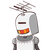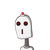# a) A, B and C contested for an election. The total number of votes were 7595394. Findivotes polled for candidate C if A and

a) A, B and C contested for an election. The total number of votes were 7595394. Findi
votes polled for candidate C if A and B got 2395710 and 321001 votes, respective
,
Who won the election and by how many votes?​

### 2 thoughts on “a) A, B and C contested for an election. The total number of votes were 7595394. Findi<br />votes polled for candidate C if A and”

1.Step-by-step explanation:

## Given:–

A, B and C contested for an election. The total number of votes were 7595394.

## To find:–

1)Find the votes polled for candidate C if A and B got 2395710 and 321001 votes, respectively?

2)Who won the election and by how many votes?

## Solution:–

Total number of votes are polled in an election = 7595394.

Number of candidates = 3

They are A , B and C

Number of votes are polled to the candidate A = 23,95,710

Number of votes are polled to the candidate B = 3,21,001

Total votes are polled to the candidates to A and B

= 23,95,710+3,21,001

=27,16,711

Total votes are polled to the candidate C

= Total votes are polled – Total votes are polled to the candidates A and B

= 75,95,394 – 27,16,711

= 48,78,683

Total votes are polled to the candidate C = 48,78,683

Candidate C won the election.

C won the election by the Candidate A and C with 48,78,683-27,16,711= 21,61,972

Candidate C won the election.

C won the election by the candidates A and B with 21,61,972 more votes.

2.Step-by-step explanation:

Given:

A , B andC contested for an election.The total votes was 7595394.

A and B got 2395710 and 321001 votes..

To find:

the votes polled for candidate C

respectively who won the election and by how much

STEP BY STEP EXPLANATION:

In the election A got = 2395710

In the election B got = 321001

Case 1 :

So, to find the vote of C

7595394 – 2395710 – 321001

= 4878683

∴ So, the vote of C in election = 4878683

Case 2 :

Who got the greatest vote in election = C

∴ So C get more vote in election is 4878683

Hence verified !